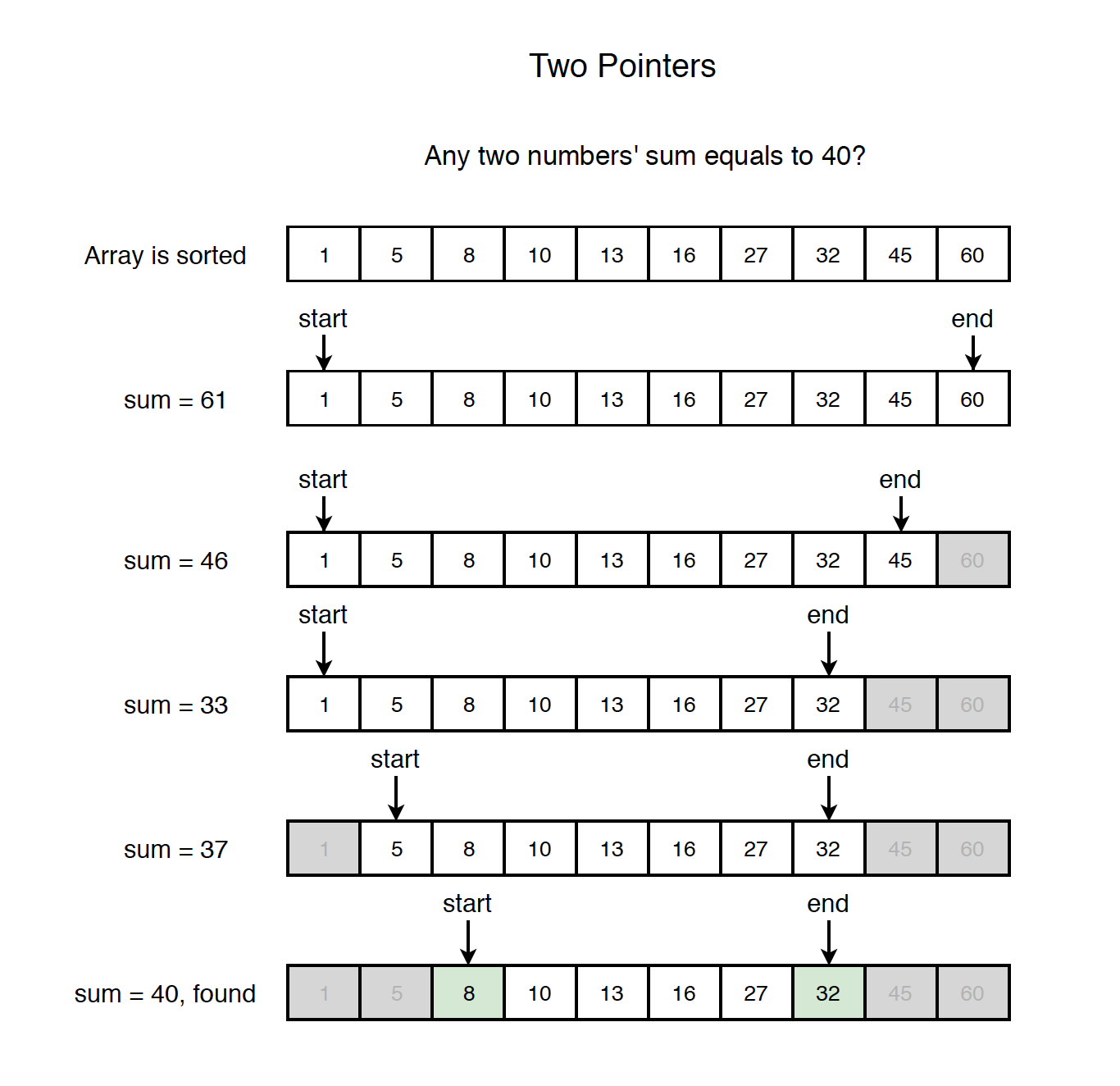# 1212. Algorithm - Two PointersTwo Pointers

Two pointers technique.

## 1. Two Pointers

Two pointers technique is easy to understand which is generally used to solve problem within linear time complexity.

### 1.1 Types of Two Pointers

• Collision - One array, move from two sides to middle.
• Forward - One array, both move forward.
• Parallel - Two arrays, each array has been assigned with a pointer.

## 2. Problem

Given a sorted (in ascending order) integer array nums of n elements and a target value, find if there exists any pair of elements (nums[i], nums[j], i!=j) such that their sum is equal to target.

### 2.1 Naive Solution

Use two loops to traverse the array to find the matched elements.

// naive solution, O(n^2)
public boolean isPairSumNaive(int[] nums, int target) {
if (nums == null || nums.length == 0) {
return false;
}

for (int i = 0; i < nums.length; i++) {
for (int j = i + 1; j <  nums.length; j++) {
if (nums[i] + nums[j] == target) {
return true; // pair exists
}

if (nums[i] + nums[j] > target)
break; // break inner loop as the array is sorted
}
}

return false;
}

• Time Complexity: $O(n^2)$

### 2.2 Two Pointers Solution

As the array is sorted, we can use two pointers to solve this problem. One pointer is initially at header, another pointer is initially at tail. Get the sum of the values represented by these two pointers. If sum is equal to target value, then return. If it is smaller than the target, move the left pointer to right; otherwise, move the right pointer to left. Thus, narrow down the scope of the candidates.See the following implementation.

// Two pointers, O(n)
public boolean isPairSum(int[] nums, int target) {
if (nums == null || nums.length == 0) {
return false;
}
int start = 0;
int end = nums.length - 1;

while (start < end) {
int sum = nums[start] + nums[end];
if (sum == target) {
return true; // pair exists
} else if (sum < target) {
start++; // move start forward to get larger value
} else {
end--; // move end backward to get smaller value
}
}

return false;
}

• Time Complexity: $O(n)$

## 3. Classic Problems

### 3.1 Collision

Sub type: Two Sum

Sub type: Partition

### 3.2 Forward

Sub type: Window

Sub type: fast and slow

### 3.3 Parallel

Two arrays, one pointer each.

Others- Todo